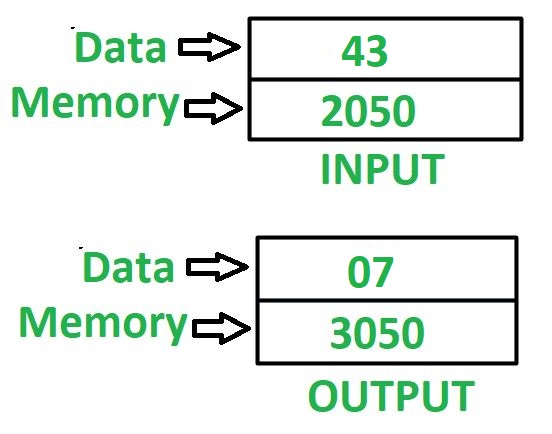# 8085 program to find sum of digits of 8 bit number

Problem – Write an assembly language program in 8085 microprocessor to find sum of digit of an 8 bit number.

Example –Assumptions – Addresses of input data and output data are 2050 and 3050 respectively.

Algorithm –

1. Load value stored at memory location 2050 in accumulator A
2. Move the value of accumulator A in register B
3. Perform masking of nibbles i.e. do AND operation of accumulator A with OF by help of ANI instruction. We will get lower nibble value in accumulator A
4. Move the value of accumulator A in register C
5. Move the value of register B in accumulator A
6. Reverse the number which is stored in accumulator A by using RLC instruction 4 times and again do masking of nibbles as done in step 3
7. Add value of register C in accumulator A
8. Store the value of A in memory location 3050

Program –

2000 LDA 2050 A <- M
2003 MOV B, A B <- A
2004 ANI 0F A <- A (AND) 0F
2006 MOV C, A C <- A
2007 MOV A, B A <- B
2008 RLC Rotate left without carry
2009 RLC Rotate left without carry
200A RLC Rotate left without carry
200B RLC Rotate left without carry
200C ANI 0F A <- A (AND) 0F
200E ADD C A <- A + C
200F STA 3050 M <- A
2012 HLT END

Explanation – Registers used A, B, C

1. LDA 2050 –loads the content of memory location 2050 in accumulator A
2. MOV B, A –moves the value of accumulator A in register B
3. ANI 0F –performs AND operation in value of accumulator A and 0F
4. MOV C, A –moves the value of accumulator A in register C
5. MOV A, B –moves the value of register B in accumulator A
6. RLC –instruction rotate the value of accumulator A, left by 1 bit. Since it is performed 4 times therefore this will reverse the number i.e swaps the lower order nibble with higher order nibble
7. Repeat step 3
8. ADD C –add the content of register of C in accumulator A
9. STA 3050 –stores value of A in 3050
10. HLT –stops executing the program and halts any further execution

My Personal Notes arrow_drop_upCheck out this Author's contributed articles.

If you like GeeksforGeeks and would like to contribute, you can also write an article using contribute.geeksforgeeks.org or mail your article to contribute@geeksforgeeks.org. See your article appearing on the GeeksforGeeks main page and help other Geeks.

Please Improve this article if you find anything incorrect by clicking on the "Improve Article" button below.